CREST Mathematics Olympiad Class 6 Sample Papers

REGISTER NOW

Syllabus:

Section 1: Knowing Our Numbers, Whole Numbers, Playing with Numbers, Basic Geometrical Ideas, Understanding Elementary Shapes, Integers, Fractions, Decimals, Data Handling, Mensuration, Algebra, Ratio And Proportion, Symmetry, Practical Geometry.

Achievers Section: Higher Order Thinking Questions - Syllabus as per Section 1

 Q.1 Fill in the blank: A quadrilateral with only one pair of opposite side parallel is called a __________
 Q.2 In the given figure ∠1 = x and ∠7 = 2x/3. Find the value of ∠5: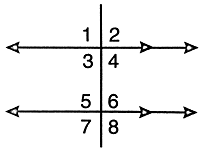Q.3 Find m and n in the given ratios:48:384 = m:784 = 53:n
 Q.4 Find the value of 'a' in the below figure, given that ROS is a straight line: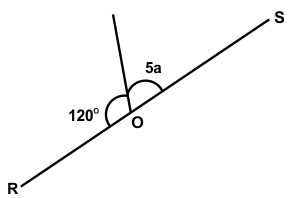Q.5 If the sum of two numbers is 55 and the H.C.F. and L.C.M. of these numbers are 5 and 120 respectively, then what is the sum of the reciprocals of these numbers?
 Q.6 In the given rectangle MNOP, if length is decreased by 3x, then find the new perimeter: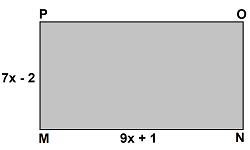Q.7 What is the product of 8/11 and the additive inverse of 44/2?
 Q.8 Annual incomes of 'A' and 'B' are in the ratio 4:5, respectively. If the income of 'A' increases by 25% and that of 'B' increases by 20%, then what is the new ratio of their incomes respectively?

Q.9

Study the bar graph and match the lists:

 List I List II (P) Total number of cars in the parking lot is 1. Blue (Q) Number of cars which are blue in color is 2. 25 (R) The least common color among the cars is 3. 70 (S) The most common color among the cars is 4. Red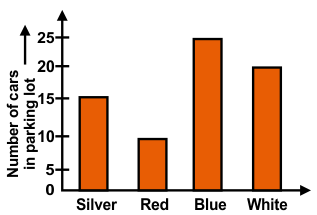Q.10 A few blocks of wood are used to make the shape of a giraffe as shown below. What is the volume of wood used to make the giraffe?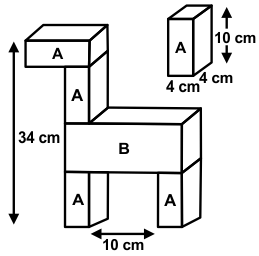Sample PDF of CREST Mathematics Olympiad for Class 6: